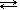# Chemistry 30

Redox Reactions: Module Index | Practice Problems | Assignments | Student Lab | Research Ideas | Teacher Resources## Acids & Bases

### 1.3 Ionization & Dissociation

You should recall from our unit on solutions that electrolytic solutions are those that conduct electricity because the substance dissolves in water to produce ions.

• Solutions that conduct electricity well are strong electrolytes – they are good conductors because they break down well and produce many ions in solution.

• Weak electrolytes do not conduct electricity as well because fewer ions are produced in solution.

This concept of strong and weak will have an important role in our discussions of acids and bases, so it is important to understand the difference now.

Ionic compounds and some molecular compounds can produce electrolytic solutions, but a different name is usually given to the processes . . .

##### Dissociation

When ionic compounds dissolve to produce ions the process is typically called dissociation.

Dissociation of ionic compounds occurs when water molecules “pull apart” the ionic crystal. This occurs due to strong attractions between the polar ends of the water molecule and the positive and negative ions within the crystal. Water molecules then surround the positive cations and negative anions; this is called hydration.

Examples of dissociation equations:

 NaCl(s) → Na+(aq) + Cl-(aq) Na2SO4 (s) → 2 Na+(aq) + SO42-(aq) (NH4)3PO4 (s) → 3 NH4+(aq) + PO43-(aq) NaOH(s) → Na+(aq) + OH– (aq) KOH(s) → K+(aq) + OH– (aq) Mg(OH)2 (s) → Mg2+(aq) + 2 OH– (aq)

What do you notice about the last three reactions shown above? All three of them produce hydroxide ions, OH . These three compounds - NaOH, KOH, and Mg(OH)2 are all Arrhrenius bases since they produce hydroxide ions in solution.

There are two important things to notice about writing dissociation equations:

1. Generally DO NOT include H2O as a reactant. We know something has been dissolved in water when we see the (aq) notation. We will make some exceptions later to this rule, however.

2. Ion charges MUST BE included!

An ion, such as the sodium ion Na+ is not the same as a sodium atom, Na. Be sure to get in the habit of writing charges for all ions; refer to your Table of Common Ions when needed, but by now you should be memorizing the charges of ions we commonly use, including polyatomic ions.

##### Ionization

When molecular compounds dissolve in water to produce ions the process is typically called ionization.

Recall that molecular compounds are held together by covalent bonding;
ionic compounds are held together by ionic bonding

Most molecular compounds do not undergo ionization. Acids are an exception. All acids produce hydrogen ions in solution. Some examples:

 HCl (g)→ H+(aq) + Cl-(aq) H2SO4 (g)→ 2 H+(aq) + SO42-(aq) HC2H3O2 (l)H+(aq) + C2H3O2-(aq) (The reason a double arrow is used for acetic acid will be discussed later)

The reactions as written above are actually simplified versions of what really occurs. Evidence suggests that the hydrogen ion, H+, actually bonds to a water molecule (H2O) to form the hydronium ion, H3O+:

 HCl(g) + H2O(l) → H3O+(aq) + Cl-(aq) H2SO4 (g) + H2O(l)→ 2 H3O+(aq) + SO42-(aq) HC2H3O2 (l) + H2O(l)H3O+(aq) + C2H3O2-(aq)

For our class, it won't make a difference if you write an acid ionization reactions as producing hydrogen ions (H+) or hydronium ions (H3O+).

For our purposes they will represent the same thing. You should be comfortable using either; one will mean the same as the other.

One last thing before we move on - on the chart on the previous page (Acids and Bases Section 1.2) a list of bases was provided along with the definition of Arrhenius acids and bases.

An Arrhenius base was defined as a substance that produces hydroxide ions in solution.

Did you notice that ammonia, NH3, does not have the required hydroxide group???

How can it be a base? (It isn't according to the Arrhenius definition, but we fully accept ammonia as a base now). There were some other problems with the Arrhenius definition as well - none that we need to dwell on, but a more general theory that dealt with the exceptions such as ammonia was needed.

Credits | Central iSchool | Sask Learning | Saskatchewan Evergreen Curriculum | Updated: 22-May-2006